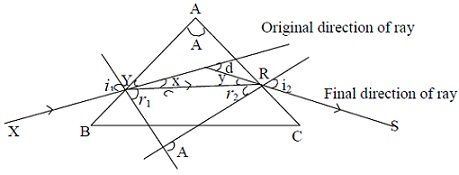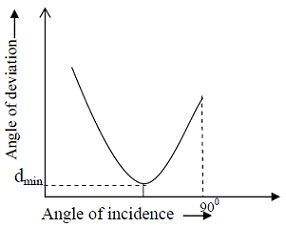#### Refraction through Prism, Physics tutorial

Angle of Deviation:

If a ray XY is incident on the face AB, it is observed that the emergent ray RS is not parallel to XY. The original ray has been deviated from its original direction by the glass prism by an angle of deviation d. The angle between the original direction and the final direction of the ray is called angle of deviation. It is denoted by d.Refer to Figure given above it can be observe that

A = r1 + r2..........................Eq.1

The angle of prism is equal to the angle between two straight lines equals to the angle between their normal. Whereas the angle of deviation can be obtained as

d = x + y (external angles of a triangle) ..........................Eq.2

But x = i1 - r1, ad y = i2 - r2 ..........................Eq.3

Hence d = (i1 - r1) + (i2 - r2) ..........................Eq.4

Minimum Deviation of the Prism:

As angle of incidence i1 is increased from 0o to 90o, deviation d decreases continuously to minimum value dmin and then increases to maximum value when i1 is 90o. This is shown in figure given below.At minimum deviation light passes symmetrically through prism

i.e. i1 = i2 and r1 = r2

Then Eq. 1 becomes

A = r1 + r2 = r + r = 2r

A = 2r....................................Eq.5

Also we know from Eq. 4, that is

d = i1 - r1 + i2 - r2

⇒ dmin = i - r + i - r = 2i - 2r

dmin = 2i - A ..........................Eq.6

From Eq.5, we can write

r = A/2..........................Eq.7

From eq.6, we can write,

i = (A + dmin)/2..........................Eq.8

Using the expression for refractive index

μ = sin i/sin r..........................Eq.9

Substituting the values of r and i from Eq.7 and Eq.8 in Eq. 9, we get the expression for refractive index

μ = (sin(A + dmin)/2)/(sin(a/2)) ..........................Eq.10

Eq. 10 is an expression the refractive index in terms of minimum deviation and refracting angle A.

As i1 = i2 at minimum deviation, it signifies that minimum deviation value is for only one angle of incidence

Maximum Deviation of the Prism:

The maximum deviation of the prism takes place when angle of incidence is 90o. Unlike minimum deviation, maximum deviation takes place for two angles of incidence namely 90o and i.

Grazing Incidence:

When i = 90°, the incident ray grazes along surface of prism and angle of refraction inside prism becomes equal to critical angle for glass - air. This is called as grazing incidence.

As A = r1 + r2, it is hence follows that at limiting angle:

A = 2C ..........................Eq.11

Grazing Emergence:

When e = 90°, emergent ray grazes along prism surface. This takes place when light ray strikes second face of the prism at critical angle for glass - air. This is called as grazing emergence.

Deviation by a Small Angle Prism:

If the refracting angle of a prism is very small, the angle of incidence i1 in most cases would also be small which implies that the incident ray would be normal at the point of entry of the prism. r1 is always smaller than i1. It also follows that r1 is small.

And sin Θ ≡ Θ(for any small angle Θ)

Therefore the refractive index μ = sin i/sin r = i1/r1

μ ≡ i1/r1

Or i1 ≡ μr1

Similarly i2 ≡ μr2

d = (i1 - r1) + (i2- r2)

d = μr1 -r1 + μr2 -r2

d = μ(r1 + r2) - (r1 + r2)

On rewriting above equation, we get

d = (μ - 1)(r1 + r2)

A = r1 + r2

Therefore d = (μ -1)A..........................Eq.12

Equation 12 states that for small angle prism deviation is independent of size of the angle of incidence. This signifies that all rays emerging from prism are deviated by same amount. This principle must be applied to lenses.

Tutorsglobe: A way to secure high grade in your curriculum (Online Tutoring)